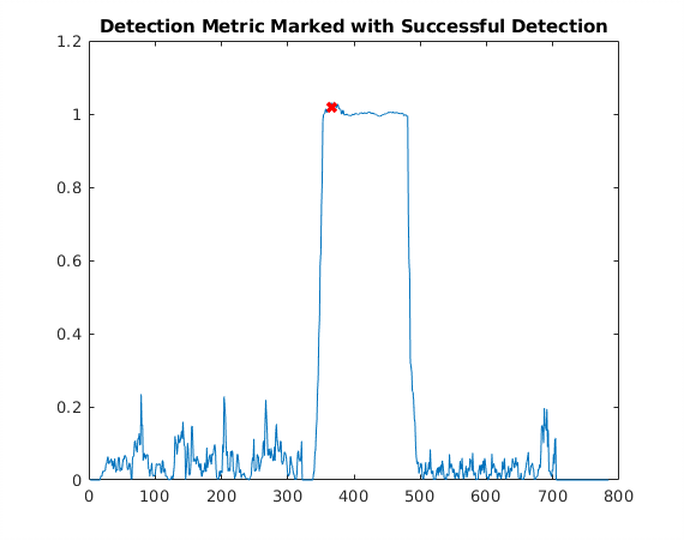HardwareTeams.com - The #1 job board and blog for electrical and computer engineers

# OFDM Timing and Synchronization Method #

This code covers: Timing and Synchronization of OFDM Modems.

OFDM has become a fundamental modulation technique in modern wireless communication systems. It is extensively used in wireless communication systems, such as Wi-Fi (IEEE 802.11a/g/n/ac/ax), 4G/LTE, and 5G networks. It offers efficient spectrum utilization and robustness against multipath fading. However, accurate synchronization of OFDM signals is critical for reliable reception and decoding.

The Schmidl and Cox Algorithm is one solution, enabling precise timing and frequency synchronization in OFDM systems.

## Papers #

Paper

OFDM Preamble Description

## Results From Code Below ### Code #

``````close all
clear all

BW = 1e7;

%parameters for sim
%carrier frequency offset
cfo = 1000/BW;

%noise amp
n_amp =.01;

%phase offset
cpo = 0;

%channel

chan = [1 .2+0.1i*.02 zeros(1,32) .05 .001+.005j];
%fft length
N=64;

%cyclic prefix length
cycPre = 16;

sw1 = sqrt(13/6)*[zeros(1,6) 0, 0, 1+j, 0, 0, 0, -1-j, 0, 0, 0, 1+j, 0, 0, 0, -1-j, 0, 0, 0, -1-j, 0, 0, 0, 1+j, 0, 0, 0, 0, 0,0, 0, -1-j, 0, 0, 0, -1-j, 0, 0, 0, 1+j, 0, 0, 0, 1+j, 0, 0, 0, 1+j, 0, 0, 0, 1+j, 0, 0 zeros(1,5)];
sw2 = [zeros(1,6) 1, 1, -1, -1, 1, 1, -1, 1, -1, 1, 1, 1, 1, 1, 1, -1, -1, 1, 1, -1, 1, -1, 1, 1, 1, 1, 0, 1, -1, -1, 1, 1, -1, 1, -1, 1, -1, -1, -1, -1, -1, 1, 1, -1, -1, 1, -1, 1, -1, 1, 1, 1, 1 zeros(1,5)];

%CREATE SHORT SYNC WORD (SYNCWORD1)
pre1 = ifft(sw1,N);
syncword1= [pre1(33:64) pre1 pre1]; %CYCLIC PREFIX

%CREATE LONG SYNC WORD (SYNCWORD2)
pre2 = ifft(sw2,N);
syncword2 = [pre2(33:64) pre2 pre2]; %CYCLIC PREFIX

%CREATE RANDOM QPSK DATA TO SEND
qpsk1 = (floor(2*rand(1,26))-.5)/.5 + 1j*(floor(2*rand(1,26))-.5)/.5;
qpsk2 = (floor(2*rand(1,26))-.5)/.5 + 1j*(floor(2*rand(1,26))-.5)/.5;
inputiFFT = [zeros(1,6), qpsk1, 0, qpsk2, zeros(1,5)];
outputiFFT = ifft((inputiFFT),N);
outputiFFT_with_CP = [outputiFFT(49:64) outputiFFT]; %CYCLIC PREFIX

%construct the signal around some noise
tx = [syncword1 syncword2 outputiFFT_with_CP];
sig = zeros(1,160);
txall = [sig sig  tx zeros(1,64)] ;
noise =n_amp*(randn(1,length(txall))+1j*randn(1,length(txall)));
IQ = filter(chan,1,txall) + noise;
nn = [0:length(IQ)-1];

w = 2*pi*cfo;
ejwn = exp(1i*(w*nn + cpo));
%apply cfo to the signal
IQ = (IQ).*ejwn;

%APPLY IQ IMBALANCE
%IQ = iqimbal(IQ,2);

figure
plot(real(IQ))

packetFound = 0;
C=zeros(1,length(IQ));
P=zeros(1,length(IQ));
M=zeros(1,length(IQ));
L = 16;
packetFindReg     = zeros(1,L);
packetFindRegDly = zeros(1,L);
detectCnt = 0;

for n= 1:length(IQ)-80

%DETECT PACKET
%paper: bottom of Matlabs page https://www.mathworks.com/help/wlan/ref/wlanpacketdetect.html
%book : practical implementaiton of OFDM systems
C(n) = conj(packetFindReg) * packetFindRegDly.';
P(n) = packetFindRegDly*packetFindRegDly';

M(n) = (abs(C(n))^2)/(P(n)*P(n));
if(M(n) > .8 & M(n) <1.2)

detectCnt = detectCnt + 1;
if(detectCnt == 16)
nPacketDetected = n;
packetFound =1;
end

else
detectCnt = 0;
end

%update registers
packetFindReg     = [packetFindReg(2:end) packetFindRegDly(1)];
packetFindRegDly  = [packetFindRegDly(2:end) IQ(n)];
end

figure
plot(M)
hold on
plot(nPacketDetected, M(nPacketDetected), 'rX', 'linewidth', 3)
title('Detection Metric Marked with Successful Detection')
``````